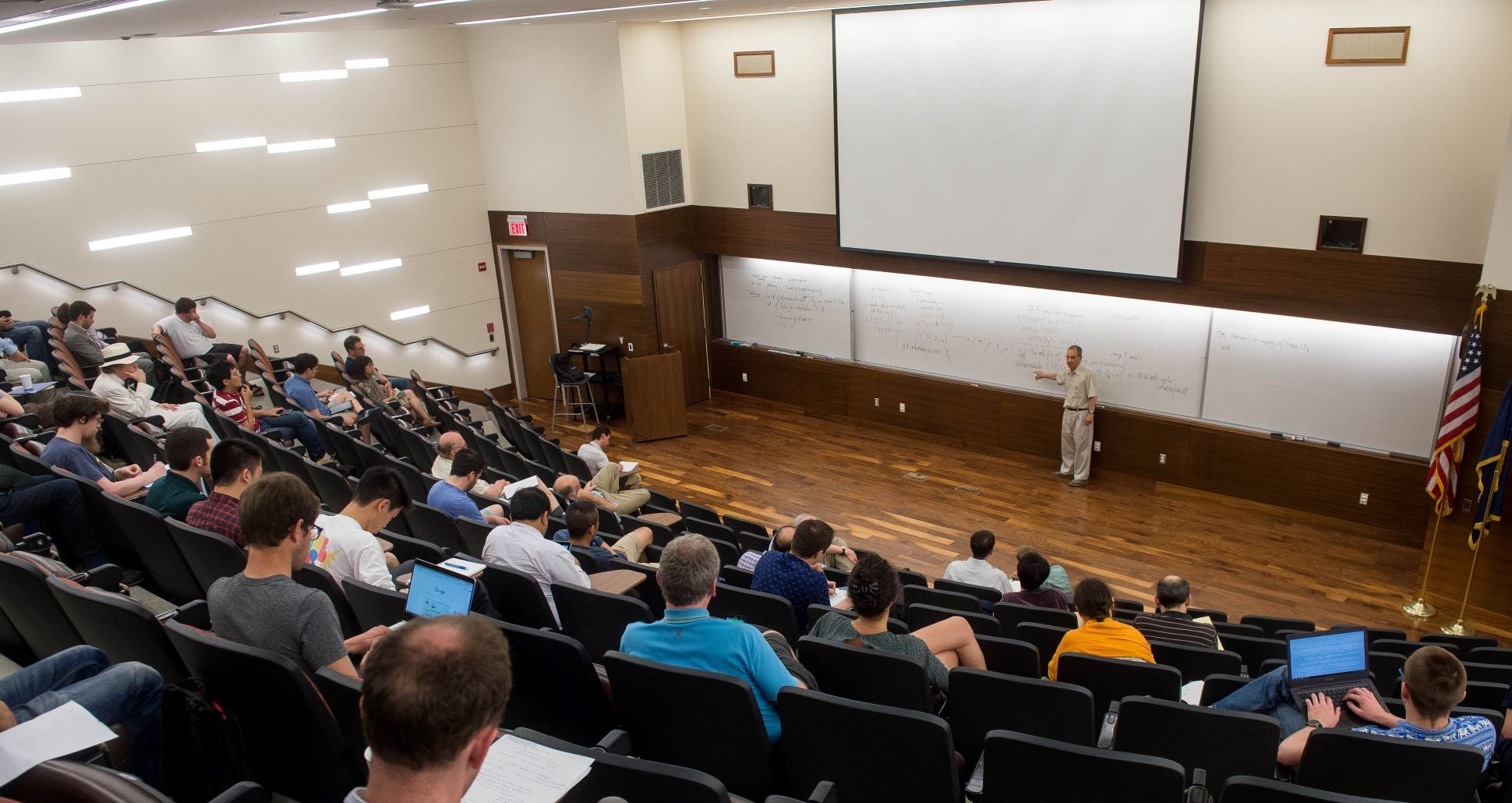Research AreasGraduate students in the Department of Mathematics will participate in many of these research areas. The faculty encourage graduate students to contact them to discuss their ideas.

Algebra, Combinatorics, and Geometry

Algebra, combinatorics, and geometry are areas of very active research at the University of Pittsburgh.

Analysis and Partial Differential Equations

The research of the analysis group covers functional analysis, harmonic analysis, several complex variables, partial differential equations, and analysis on metric and Carnot-Caratheodory spaces.

Applied Analysis

The department is a leader in the analysis of systems of nonlinear differential equations that arise in modeling a variety of physical phenomena. They include problems in biology, chemistry, phase transitions, fluid flow, flame propagation, diffusion processes, and pattern formation in nonlinear stochastic partial differential equations.

Mathematical Biology

The biological world stands as the next great frontier for mathematical modeling and analysis. This group studies complex systems and dynamics arising in various biological phenomena.

Mathematical Finance

A rapidly growing area of mathematical finance is Quantitative Behavioral Finance. The high-tech boom and bust of the late 1990s followed by the housing and financial upheavals of 2008 have made a convincing case for the necessity of adopting broader assumptions in finance.

Numerical Analysis and Scientific Computing

The diversity of this group is reflected in its research interests: numerical analysis of partial differential equations, adaptive methods for scientific computing, computational methods of fluid dynamics and turbulence, numerical solution of nonlinear problems arising from porous media flow and transport, optimal control, and simulation of stochastic reaction diffusion systems.

Topology and Differential Geometry

Research in analytic topology continues in the broad area of generalized metric spaces. This group studies relativity theory and differential geometry, with emphasis on twistor methods. As well as, geometric and topological aspects of quantum field theory, string theory, and M-theory.